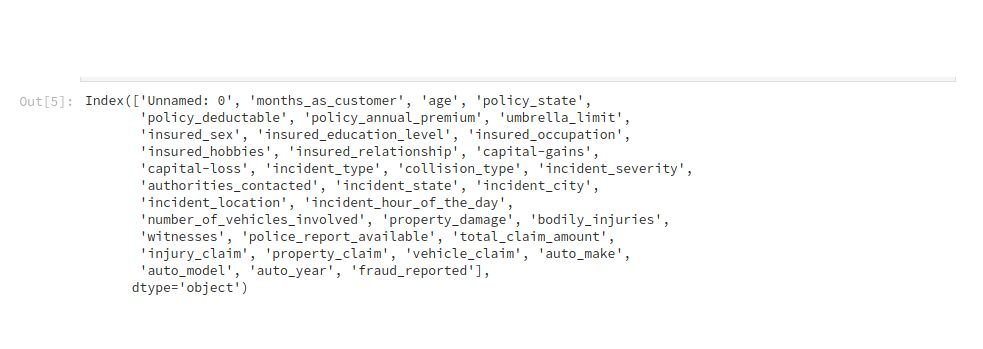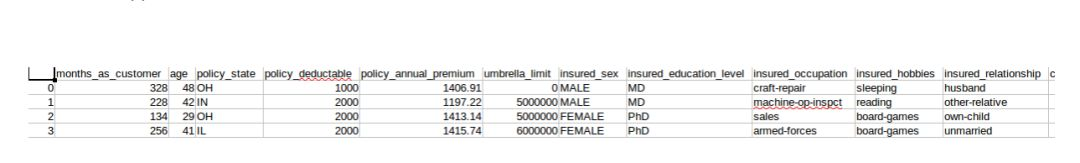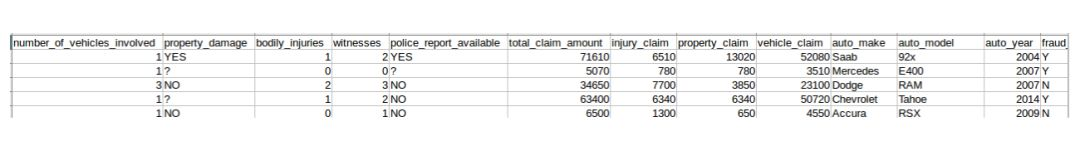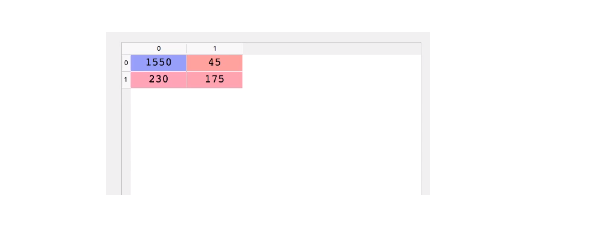>科技>正文

# 【DS】Keras深度学习介绍

1 您使用过哪些深度学习框架？

2 您用过Keras吗？您用Keras解决什么问题？2importnumpy asnp

1feats= [ 'policy_state', 'insured_sex', 'insured_education_level', 'insured_occupation', 'insured_hobbies', 'insured_relationship', 'collision_type', 'incident_severity', 'authorities_contacted', 'incident_state', 'incident_city', 'incident_location', 'property_damage', 'police_report_available', 'auto_make', 'auto_model', 'fraud_reported', 'incident_type']

2

3df_final= pd.get_dummies(df, columns=feats, drop_first= True)

1fromsklearn.model_selection importtrain_test_split

1X= df_final.drop([ 'fraud_reported_Y', 'policy_csl', 'policy_bind_date', 'incident_date'],axis= 1).values

2y= df_final[ 'fraud_reported_Y'].values

1X_train, X_test, y_train, y_test = train_test_split(X, y, test_size=0.3)

1from sklearn.preprocessing import StandardScaler

2sc = StandardScaler()

3X_train = sc.fit_transform(X_train)

4X_test = sc.transform(X_test)

1importkeras

1fromkeras.models importSequential

2fromkeras.layers importDense

1classifier= Sequential()

2Dense( 3, kernel_initializer = ‘uniform’,

3activation = ‘relu’, input_dim= 5))

2Dense( 3, kernel_initializer = ‘uniform’,

3activation = ‘relu’))

2Dense( 1, kernel_initializer = ‘uniform’,

3activation = ‘sigmoid’))

2loss = ‘binary_crossentropy’,

3metrics = [‘accuracy’])

X_train表示我们用来训练ANN的自变量，y_train表示我们预测的列。Epochs表示通过ANN传递完整数据集的次数。Batch_size是权重将被更新的观察值的数量。

1y_pred= (y_pred > 0.5)

2cm = confusion_matrix(y_test, y_pred)1new_pred = classifier.predict(sc.transform(np.array( [[a,b,c,d]])))

1new_pred= (new_prediction > 0.5)

Keras有一个scikit学习包装器(KerasClassifier)，它允许我们在Keras代码中包含K-fold交叉验证。

1fromkeras.wrappers.scikit_learn importKerasClassifier

1fromsklearn.model_selection importcross_val_score

KerasClassifier希望它的一个参数是一个函数，因此我们需要构建这个函数。这个函数的目的是构建我们的ANN的架构。

1def make_classifier():

2classifier = Sequential()

3classiifier. add(Dense( 3, kernel_initializer = ‘uniform’, activation = ‘relu’, input_dim= 5))

4classiifier. add(Dense( 3, kernel_initializer = ‘uniform’, activation = ‘relu’))

5classifier. add(Dense( 1, kernel_initializer = ‘uniform’, activation = ‘sigmoid’))

6classifier.compile(optimizer= ‘adam’,loss = ‘binary_crossentropy’,metrics = [‘accuracy’])

7returnclassifier

1classiifier = KerasClassifier(build_fn = make_classifier,

2batch_size=10, nb_epoch=100)

1accuracies = cross_val_score(estimator = classifier,

2X = X_train,

3y = y_train,

4cv = 10,

5n_jobs = -1)

1mean= accuracies.mean()

1variance= accuracies.var()

1fromkeras.layers import Dropout

2

3classifier = Sequential()

4classiifier. add(Dense( 3, kernel_initializer = ‘uniform’, activation = ‘relu’, input_dim= 5))

5

6# Notice the dropouts

8classiifier. add(Dense( 6, kernel_initializer = ‘uniform’, activation = ‘relu’))

10

11classifier. add(Dense( 1, kernel_initializer = ‘uniform’, activation = ‘sigmoid’))

12classifier.compile(optimizer= ‘adam’,loss = ‘binary_crossentropy’,metrics = [‘accuracy’])

1fromsklearn.model_selection importGridSearchCV

1def make_classifier(optimizer):

2classifier = Sequential()

3classiifier. add(Dense( 6, kernel_initializer = ‘uniform’, activation = ‘relu’, input_dim= 11))

4classiifier. add(Dense( 6, kernel_initializer = ‘uniform’, activation = ‘relu’))

5classifier. add(Dense( 1, kernel_initializer = ‘uniform’, activation = ‘sigmoid’))

6classifier.compile(optimizer= optimizer,loss = ‘binary_crossentropy’,metrics = [‘accuracy’])

7returnclassifier

1classifier= KerasClassifier(build_fn = make_classifier)

1params= {

2'batch_size':[ 20, 35],

3'nb_epoch':[ 150, 500],

5}

1grid_search = GridSearchCV(estimator=classifier,

2param_grid=params,

3scoring=’accuracy’,

4cv=10)

1grid_search= grid_search.fit(X_train,y_train)

1best_param= grid_search.best_params_

2best_accuracy= grid_search.best_score_

Cheers.